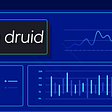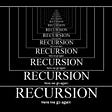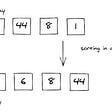# What’s all the fuss about?

Recursion seems to be the one topic that gives every beginning computer science student a headache for months. This concept can be introduced through a variety of different materials, however, it is most notably seen in the form of recursive functions. Functions, in general, are used to group statements together that perform a specific task and to get rid of repetitive lines of code. You call a function to execute lines of code. Functions can even call other functions to assist in completing the process at hand. A function that calls itself is referred to as a recursive function.

# Key terms to know

• Base Case
• Recurrence Relation
• Recursive Definition
• Recursive Descent
• Recursive Ascent

The base case is essentially the simplest form of the problem. We need a base case within our recursive implementations to prevent the function from calling itself infinitely.

The recurrence relation is the use of defining the problem in terms of a simpler version of itself.

The combination of both the base case and recurrence relation gives us the recursive definition. Recursive implementations almost always come directly from the recursive definition.

Recursive descent is when a function continuously calls itself until a base case is reached.

Recursive ascent is the return to each recursive function call when the base case is reached.

# Explaining recursion through the factorial of a number

In the image above, you can see how each of the key terms can be linked to some part of the process for computing/implementing the factorial of a number. The factorial of a number can be written as the number multiplied by the factorial of the number minus one. Showing the process that 4! = 4* 3!, 3! = 3 * 2!, and so on, results in the recursive descent. Once 0! has been hit, replacing it with 1 allows us to start computing the factorial. The successive computation that results from completing the multiplication of each factorization results in the recursive ascent. The factorial of 0 being 1 is our base case (the simplest form of the problem). 4! being equal to 4 * (4 - 1)! is our recurrence relation (the problem being defined in terms of a simpler version of itself). A more general recurrence relation for the factorial of any number would be n! = n * (n - 1)!. For any recursive implementation, we always want to start by writing the base case first. By doing this, it ensures that our function will not perform recursion infinitely (assuming that our base case and recurrence relation are correct). As you can see in the image above, the base case and recurrence relation being written together form the recursive definition. The recursive definition will allow us to derive the implementation for computing the factorial of a number.

# Recursive factorial implementation

## Alternative implementation

Note: The base case and recurrence relation are variable. Their values are entirely dependent on the problem you are trying to solve recursively. Before trying to solve any problem recursively, you must determine the correct base case and recurrence relation. However, the saying goes something like: “Any problem that can be solved recursively can be solved iteratively.” Therefore, do not try to solve everything recursively. If something can be solved iteratively, then solve it iteratively (unless recursion produces quicker run-time speeds).

# A basic explanation of the linear linked list data structure

Now, the only reason why I am even mentioning linear link lists in an article on recursion is because of its relationship to my method for helping to understand how recursion works. The image above depicts how a linear linked list is represented in a drawn format. The word “list” is a pointer to the first node in the linked list. A pointer is just a variable that stores the address of some item in memory. In this case, the list stores the address (in memory) of the first node in the list. Each of the boxes is called nodes. A node for a linked list consists of an info field and a text field. The info field can contain any kind of item. These items can include anything from integers to strings, and even objects. The next field contains the address for the next node in the linked list. We say that the next field “points” to the next node in the list.

Note: The last node in the image above has a strange-looking pointer that ends with two dashes. This notation indicates that the last node’s next field does not contain any address to another node. Therefore, the notation indicates the “end of the list.”

# My method for visually seeing how recursion works

How have I applied the concepts associated with a linear linked list towards understanding recursion? In the image above, you can see how each factorial function call has its own pointer. The idea is to think of every function call as having access to its own function implementation. Another way to put it is that each function call “points” to its own implementation. Thinking of recursion in this way allows you to visually see the recursive descent and recursive ascent in action. As you follow the pointers (or arrows if that helps you understand it better), going from one function implementation to the next, you are performing the recursive descent. When you get to the last function implementation (when n = 0), following the pointers backward to each function call performs the recursive ascent and ultimately computes 4!.

Note: I wrote the else statement on a single line (when n = 1) to save room. This does make the method work any better or worse. If the else statement was written on another line, it would not affect how the method is supposed to work whatsoever.

My method for visually seeing how recursion works can be applied to any recursive implementation. I have even used this method to see how the recursive implementation for the Fibonacci sequence works. Yes, writing out this process like the image above may not be suitable for every situation, but in the end, it helps you understand recursion. Understanding recursion is all that really matters.

--

--

--

## How To Automate AWS EBS Snapshot Using AWS Snapshot Lifecycle Policy## Mutual Fund Investment website created with Streamlit, hosted securely on custom domain on AWS## How Razorpay uses Druid for seamless analytics and product insights?## BASIC for the Visual Age## INTRODUCTION TO PANDAS## Joey Zamiski

Computer Science student. Hopeful Research Scientist in Computer Graphics.

## What is recursion?## RECURSION## Recursion 101 | Understanding the Basics## Sorting Algorithms: Insertion Sort